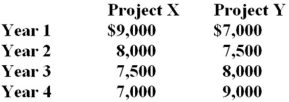# Fundamentals of Corporate Finance Study Set 23

## Quiz 6 : Bond ValuationStudy FlashcardsLooking for Finance Homework Help?

## Quiz 6 :Bond Valuation

Question TypeWhat is the interest rate charged per period multiplied by the number of periods per year called?
Free
Multiple Choice

B

Tags
Choose question tagWhich one of the following statements related to annuities and perpetuities is correct?
Free
Multiple Choice

B

Tags
Choose question tagWhich one of the following terms is defined as a loan wherein the regular payments, including both interest and principal amounts, are insufficient to retire the entire loan amount, which then must be repaid in one lump sum?
Free
Multiple Choice

C

Tags
Choose question tagWhich one of the following terms is used to describe a loan that calls for periodic interest payments and a lump sum principal payment?
Multiple Choice
Tags
Choose question tagYou are considering two projects with the following cash flows:Which of the following statements are true concerning these two projects? I.Both projects have the same future value at the end of year 4, given a positive rate of return. II.Both projects have the same future value given a zero rate of return. III.Project X has a higher present value than Project Y, given a positive discount rate. IV.Project Y has a higher present value than Project X, given a positive discount rate.
Multiple Choice
Tags
Choose question tagWhich one of the following statements concerning interest rates is correct?
Multiple Choice
Tags
Choose question tagThe interest rate that is quoted by a lender is referred to as which one of the following?
Multiple Choice
Tags
Choose question tagAn amortized loan:
Multiple Choice
Tags
Choose question tagA monthly interest rate expressed as an annual rate would be an example of which one of the following rates?
Multiple Choice
Tags
Choose question tagWhich one of the following compounding periods will yield the smallest present value given a stated future value and annual percentage rate?
Multiple Choice
Tags
Choose question tagHow is the principal amount of an interest-only loan repaid?
Multiple Choice
Tags
Choose question tagWhich one of these statements related to growing annuities and perpetuities is correct?
Multiple Choice
Tags
Choose question tagAn ordinary annuity is best defined by which one of the following?
Multiple Choice
Tags
Choose question tagWhich one of the following statements correctly states a relationship?
Multiple Choice
Tags
Choose question tagWhich of the following statements related to interest rates are correct? I.Annual interest rates consider the effect of interest earned on reinvested interest payments. II.When comparing loans, you should compare the effective annual rates. III.Lenders are required by law to disclose the effective annual rate of a loan to prospective borrowers. IV.Annual and effective interest rates are equal when interest is compounded annually.
Multiple Choice
Tags
Choose question tagA loan where the borrower receives money today and repays a single lump sum on a future date is called a(n) _____ loan.
Multiple Choice
Tags
Choose question tagWhich one of the following terms is used to describe a loan wherein each payment is equal in amount and includes both interest and principal?
Multiple Choice
Tags
Choose question tagThe entire repayment of which one of the following loans is computed simply by computing a single future value?
Multiple Choice
Tags
Choose question tagWhich one of the following accurately defines a perpetuity?
Multiple Choice